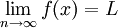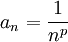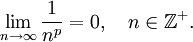# Relationship to Limits of Functions

Previous: Convergence of Infinite Sequences Example

Next: Limit Laws for Infinite Sequences

Our definition of convergence for an infinite sequence may look like the definition of a limit for functions.

Theorem: Relation to Limits of Functions

If we have thatand f(n) = an, where x is a real number and n is an integer, thenThe above theorem makes it easier for us to evaluate limits of sequences.

## Simple Example

Suppose we wish to determine whether the sequenceconverges, where p is any positive integer.

Since we know thatfor any integer p greater than zero, our theorem yieldsTherefore, the given sequenceconverges.

Previous: Convergence of Infinite Sequences Example

Next: Limit Laws for Infinite Sequences